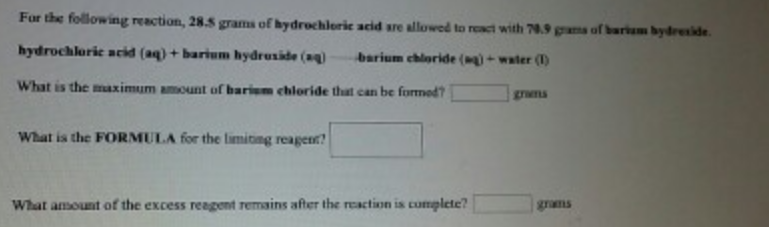# Problem: For the following reaction, 28.5 grams of hydrochloric acid are allowed to react with 70.9 grams of barium hydroxide.hydrochloric acid (aq) + barium hydroxide (aq) → barium chloride (aq) + water (l) What is the maximum amount of barium chloride that can be formed?What is the FORMULA for the limiting reagent? What amount of the excess reagent remains after the reaction is complete?

###### FREE Expert Solution
79% (144 ratings)###### Problem Details

For the following reaction, 28.5 grams of hydrochloric acid are allowed to react with 70.9 grams of barium hydroxide.

hydrochloric acid (aq) + barium hydroxide (aq) → barium chloride (aq) + water (l)

What is the maximum amount of barium chloride that can be formed?

What is the FORMULA for the limiting reagent?

What amount of the excess reagent remains after the reaction is complete?